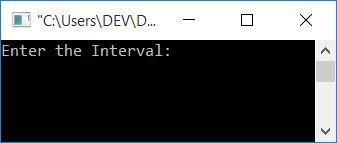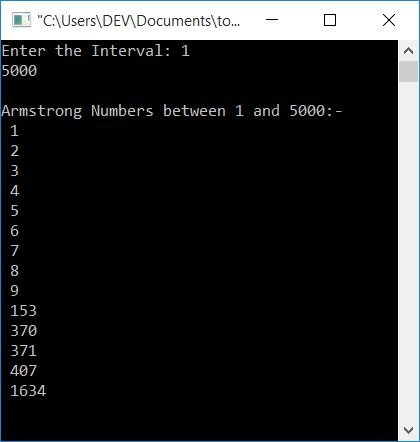# C Program to Generate Armstrong Numbers

In this article, you will learn and get code to print Armstrong numbers in a given range with and without using a user-defined function. But before going through the program, let's first understand it.

### What is the Armstrong number?

An Armstrong number is a number that equals the sum of its own digits, where each digit is raised to the power of the number of digits. For example, 153 is an Armstrong number because:

```153 = 13+53+33
= 1+125+27
= 153```

Since 153 is a 3-digit number, each digit is raised to the power of 3. Now let's move on and implement it in a C program.

## Generate Armstrong Numbers within the Given Range

This program prints all the Armstrong numbers that lie within the range provided by the user at run-time.

```#include<stdio.h>
#include<conio.h>
int main()
{
int start, end, temp, noOfDigit, num, res=0, rem, pow, i;
printf("Enter the Interval: ");
scanf("%d%d", &start, &end);
printf("\nArmstrong Numbers between %d and %d:-\n", start, end);
while(start<=end)
{
temp = start;
noOfDigit=0;
while(temp>0)
{
temp = temp/10;
noOfDigit++;
}
num = start;
while(num>0)
{
rem = num%10;
pow = 1;
i = 0;
while(i<noOfDigit)
{
pow = pow*rem;
i++;
}
res = res + pow;
num = num/10;
}
if(res==start)
printf(" %d\n", res);
start++;
res = 0;
}
getch();
return 0;
}```

This program was built and runs under the Code::Blocks IDE. Here is its sample run:Now supply two numbers as intervals, say 1 and 5000, to print all the Armstrong numbers between these two. That is, the snapshot given below shows all the Armstrong numbers from 1 to 5000:Note: Every single-digit number is an Armstrong number. Since it is a 1-digit, therefore, the power raised to it will be 1 (as defined in the definition given above), and any number raised to the power of 1 is equal to the number itself.

Therefore, 1, 2, 3, 4, 5, 6, 7, 8, 9, 153, 370, 371, 407, and 1634 are Armstrong numbers between 1 and 5000. Because,

• 1 = 11 (digit to the power of 1, for 1-digit number)
• 2 = 21
• 3 = 31
• 4 = 41
• 5 = 51
• 6 = 61
• 7 = 71
• 8 = 81
• 9 = 91
• 153 = 13+53+33 (digit to the power of 3, for 3-digit number)
• 370 = 33+73+03
• 371 = 33+73+13
• 407 = 43+03+73
• 1634 = 14+64+34+44 (digit to the power of 4, for 4-digit number)

The following block of code

```temp = start;
noOfDigit=0;
while(temp>0)
{
temp = temp/10;
noOfDigit++;
}```

is used to find the number of digits in the current number. That is, the value of the start variable gets initialized to temp, and using this variable, this block of code finds how many digits this number has.

Here is another block of code,

```pow = 1;
i = 0;
while(i<noOfDigit)
{
pow = pow*rem;
i++;
}```

which is used to find the digit raised to the power's value. For example, if the current digit is 3 and the number is a 3-digit number, then this block of code will find the value of 33. Now let's create the same-purpose program using a user-defined function.

### Generate Armstrong numbers using a user-defined function

This program is easy to understand as it was created using user-defined functions. That is, the two blocks of codes that were required to run more than once are shifted in the function, and the names of those two functions are findNoOfDigit(int) (finds number of digits) and pow(int rem, int noOfDigit) (finds digit raised to power's value). Finding the number of digits means that if a number is 370, it will therefore return 3. because it is a 3-digit number. Finding a digit raised to the power's value means that if a digit is 3 (from a 3-digit number), then 33 equals 27, so function returns 27.

```#include<stdio.h>
#include<conio.h>
int findNoOfDigit(int);
int pow(int, int);
void generateArmNos(int, int);
int main()
{
int start, end;
printf("Enter the Interval: ");
scanf("%d%d", &start, &end);
printf("\nArmstrong Numbers between %d and %d:-\n", start, end);
generateArmNos(start, end);
getch();
return 0;
}
void generateArmNos(int start, int end)
{
int noOfDigit, num, digToPowVal, i, rem, res=0;
while(start<=end)
{
noOfDigit = findNoOfDigit(start);
num = start;
while(num>0)
{
rem = num%10;
digToPowVal = pow(rem, noOfDigit);
res = res+digToPowVal;
num = num/10;
}
if(res==start)
printf(" %d\n", res);
start++;
res = 0;
}
}
int findNoOfDigit(int temp)
{
int noOfDigit=0;
while(temp>0)
{
temp = temp/10;
noOfDigit++;
}
return noOfDigit;
}
int pow(int rem, int noOfDigit)
{
int digToPowVal = 1, i=0;
while(i<noOfDigit)
{
digToPowVal = digToPowVal*rem;
i++;
}
return digToPowVal;
}```

This program produced the same output as the previous one.

#### The same program in different languages

C Quiz

« Previous Program Next Program »